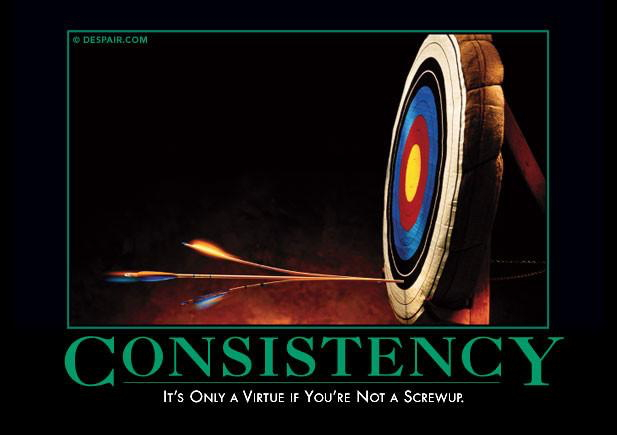## Blog Entries#### Accuracy and Precision

Accuracy and Precision
Sometimes in science we use words that you think you know, but we have more specific meanings for them. For example, most people think that "velocity" and "speed" mean the same thing. If you had physics with us, however, you should know that they are not (velocity includes not only speed, but direction as well). Accuracy and precision is another case like that. Most people think of them as meaning the same thing, but they really are quite different. Accuracy is how close a measurement comes to the correct result. This assumes of course, that you know the correct result. Usuall, you don't; so it is not always possible to determine accuracy. Here is a link to the Wikipedia entry on the age of the Earth. I would like you to read the opening 2 sections (through “early calculations”).
Precision is how close all of your measurements are to each other. You can always determine the precision of measurements by repeating them several times. Increased precision corresponds to an increased number of significant figures. How would you determine the ACCURACY of a balance? To do this, you would have to have something that is a known standard mass (these can be purchased). All this is an object that has been made to very high precision to be a mass we have agreed upon as “standard.” You would determine it's mass with your balance and see if the result you get is the same as the accepted mass. You might want to take ten measurements of the standard mass and record each. Then you could take the average measurement and compare it to the accepted amount. You might find that the balance was consistently off in one direction. Suppose a 20.00g standard mass always comes out between 19.20 and 19.45. You could say that the instrument is consistently off by between -.80 to -.55g. So it is consistently under mass on average by -.0675. However, there is an interesting significant figure question about how far I should take this. Should I report -0.675 or round it to -0.68? In practice, you would calibrate the balance to read the correct mass. Alternatively, you could simply add the .068 to every measurement. This is similar to what you would do if you knew your clock always ran 5 minutes fast...you would just take off 5 minutes to get the correct time (which, again can only be stated because we have agreed upon standards). How would you determine the PRECISION of a balance? Let's look at those ten measurements again. The range of the answers is between 19.20 and 19.45g. The range is .25g, or +/-0.125g. In practice, we would say that this balance is precise to the tenths of a gram. This would not be an especially precise balance. ours should give us variation of less than 0.1g. Usually, we expect a well maintained instrument to show variation only in the last digit to which it gives information. So, if the balance shows results to the hundredths place, we expect the precision to be in that range. This is analogous to a ruler marked to the nearest millimeter. We expect that we can consistently determine length to the nearest mm, and then estimate the next digit. The last digit of any measurement is soft. That is, it is always an estimate. You will notice this when you use the balance. As a result, any calculations based on this will also be limited by the precision of the actual measurement.
Discussion questions: Which would you rather have: a balance that is imprecise, or one that is precise, but consistently off by a known amount? Often, we see political polls reported +/- X%, let's say 2%. Is that precision or accuracy? Hint: does anyone know what the correct answer is? Significant figures and precision It may be obvious to you from the discussion above that the significant figures with which I report a measurement and its units essentially tell you the precision. If my balance reports a number in the 0.001g place (milligram), I can say that it is precise to number of milligrams.